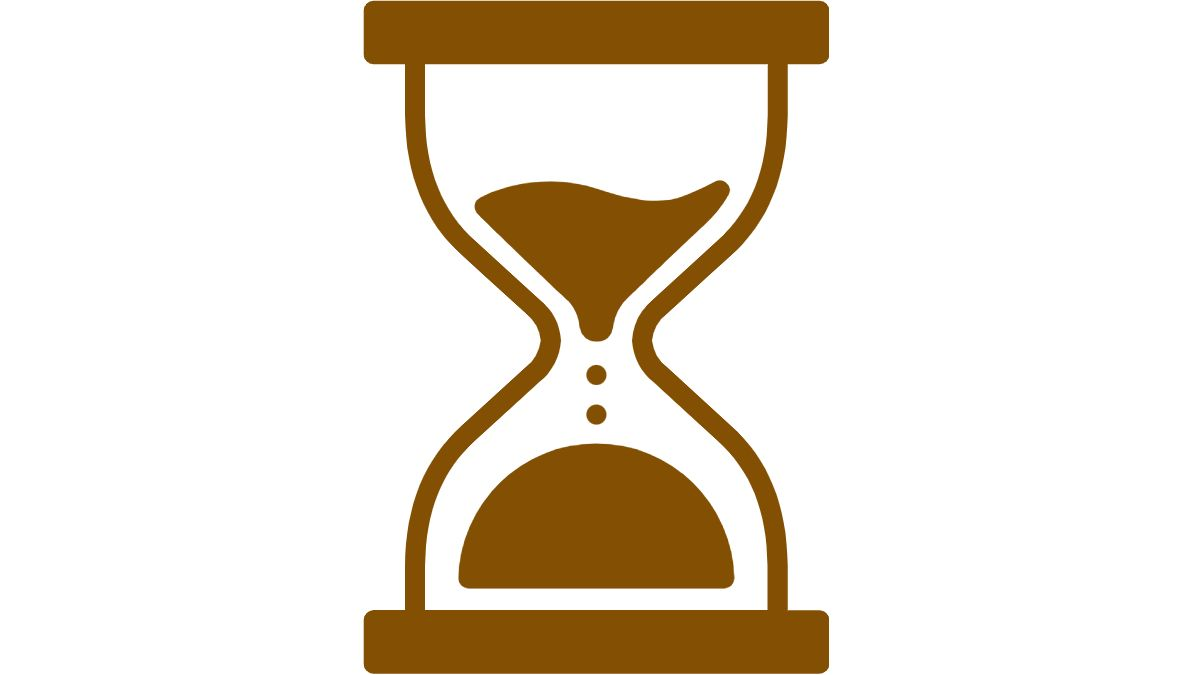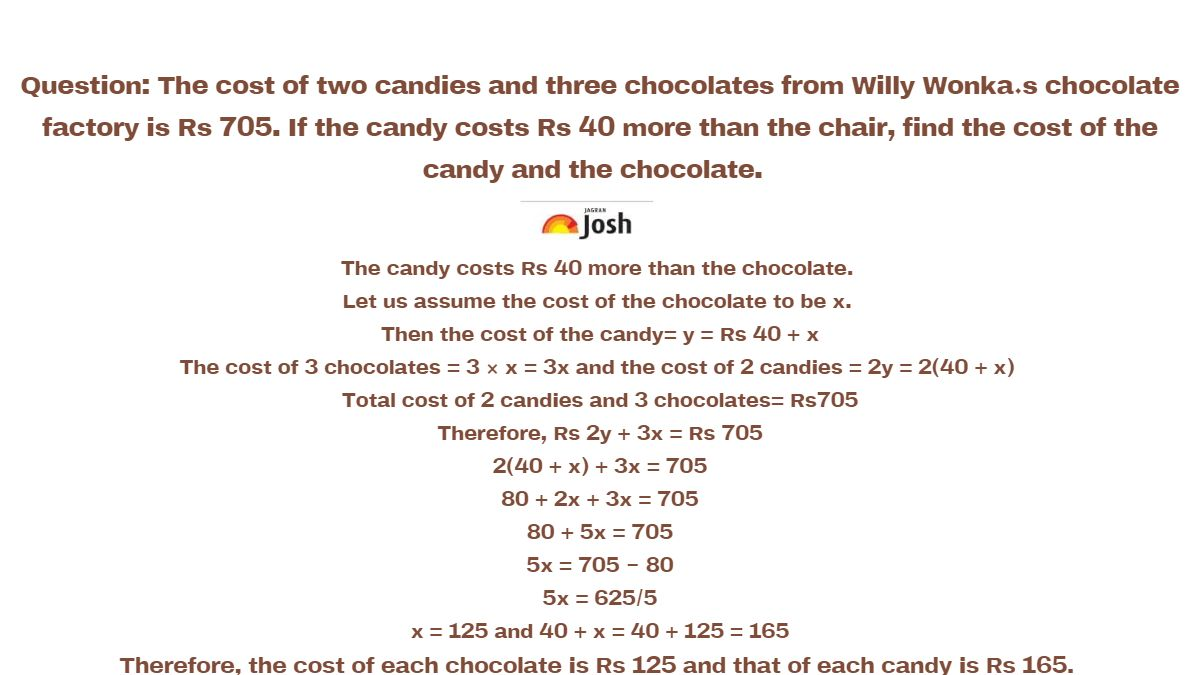# Can you Help Riya Buy Willy Wonka’s Chocolates by Solving This Question

Maths Riddle for School Kids: Little Riya is hungry and wants to eat her favourite chocolate. Can you help Riya buy her chocolate from Willy Wonka’s chocolate factory by solving this Maths puzzle for school students?

Tricky Maths Riddles for School Students: Little Riya is hungry and stuck in Willy Wonka’s chocolate factory. She is smart for her young age and tried to elope, but all her efforts failed in front of the cunning chocolate villain. She is too young to catch hold of Willy Wonka’s vicious games. But she will be set free and given lots of her favourite chocolate from the factory IF you can help her solve a maths puzzle. Can you help Riya in getting her favourite chocolate?

Are You Ready to Help Riya?

 The cost of two candies and three chocolates from Willy Wonka’s chocolate factory is Rs 705. If the candy costs Rs 40 more than the chocolate, find the cost of the candy and the chocolate.Oh! Did you not know that you have only 30 seconds to solve this Maths Riddle for School Kids?Do not be scared. Willy Wonka is scary looking but you can do it!

Read the question carefully and make a note of the details given.

 Here’s some help: What is the total cost of the candies and chocolates? Which is more expensive: candy or chocolate?

HINT:

Try to remember your chapter notes from CBSE Class 10 Maths Chapter Linear Equation in Two Variables

The clock is ticking!

See the two variables here are the price of candies and chocolates.

You have 18 more seconds to solve the question!

TICK TICK

TICK TICKTime is flying, quite literally!

Help little Riya in escaping and get her favourite chocolates.

Do you need more help?

Check CBSE Class 10 Maths Linear Equations in Two Variables Mind Map

Tick

Tick

Tick

 Here’s another hint Assume the cost of the chocolate to be x.  Then the cost of the candy is y i.e Rs 40 + x

You have 10 more seconds before hungry Riya is kept in Willy Wonka’s chocolate factory forever!

Tick

Tick

Tick

And the time is over!

Were you able to get the values of X and Y. Did you get the answer?

 Cost of chocolate = Rs 125 Cost of candy = Rs 165

Check the solution below:

The candy costs Rs 40 more than the chocolate.

Let us assume the cost of the chocolate to be x.

Then the cost of the candy= y = Rs 40 + x

The cost of 3 chocolates = 3 × x = 3x and the cost of 2 candies = 2y = 2(40 + x)

Total cost of 2 candies and 3 chocolates= Rs705

Therefore, Rs 2y + 3x = Rs 705

2(40 + x) + 3x = 705

80 + 2x + 3x = 705

80 + 5x = 705

5x = 705 – 80

5x = 625/5

x = 125 and 40 + x = 40 + 125 = 165

Therefore, the cost of each chocolate is Rs 125 and that of each candy is Rs 165.ALSO CHECK:

Tricky Science Riddle: You have a Topper’s Brain if you Solve this Riddle in 10 Seconds